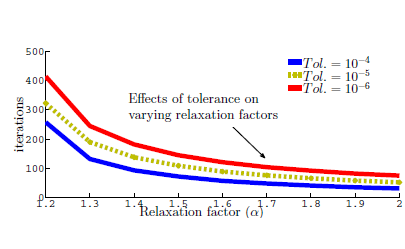# Extension of ADMMAlgorithmin Solving Optimal Control Model Governed by Partial Differential Equation

## Authors

• Kazeem adebowale Dawodu Department ofMathematical Sciences, Federal University of Technology Akure, Nigeria

## Keywords:

Optimal Control model, Alternating Direction Method of Multipliers, Partial Differential Equation constraint, Crank-Nicolson, Composite Simpson’s, Consistency and Stability

## Abstract

This paper presents an Algorithm for the numerical solution of the Optimal Control model constrained by Partial Differential Equation using the Alternating Direction Method of Multipliers (ADMM) accelerated with a parameter factor in the sense of Nesterov. The ADMM tool was
applied to a partial differential equation-governed optimization problem of the one-dimensional heat equation type. The constraint and objective functions of the optimal control model were discretized using the Crank-Nicolson and Composite Simpson’s Methods respectively into a derived discrete convex optimization form amenable to the ADMM. The primal-dual residuals were derived to ascertain the rate of convergence of themodel for increasing iterates. An existing example was used to test the efficiency and degree of accuracy of the algorithm and the results were favorable when compared the existing method.

Dimensions

J. T. Betts & S. L. Campbell Discretize then Optimize, in Mathematics for Industry: Challenges and Frontiers, SIAM (2005).

K. Ghobadi, N. Nedialkov & T. Terlaky, On the Discretize then Optimize Approach", Preprint for Industrial and Systems Engineering, 2009

O. Olotu & K. A. Dawodu, "On the Discretized Algorithm for Optimal Proportional Control Constrained by Delay Differential Equation", Journal of Mathematical Theory and Modelling 3 (2013) 157.

O. Olotu, K. A. Dawodu & T. T. Yusuf, Algorithm for Solving Multi-Delay Optimal Control

Problems Using Modified Alternating Direction Method of Multipliers. Journal of Applied & Comp. Mathematics 8 (2019) 445.

O. Olotu & K. A. Dawodu, "Numerical Solution to One –Dimensional Multi-Delay System using the Modified Alternating Direction Method of Multipliers", Journal of NAMP 50 (2019) 107.

B. He & X. Yuan, "Block-wise Alternating Direction Method of Multipliers for Multiple-block Convex Programming and Beyond. SIAM Journal of Comp. Mathematics, 691 (2015), 145-.

B. He, X. Hong & X. Yuan, "On the proximal jacobian decomposition of alm for multiple-block separable convex minimization problems and its relationship to ADMM", Journal of Scientific Computing 66 (2016) 1204.

S. Kameswaran and L. T. Beigler,Advantages of Nonlinear Programming-based Methodologies or Inequality Path Constrained Optimal Control Problem - a Numerical Study, SIAM Journal on Scientific Comp. 30 (2008) 957.

Y. E. Nesterov, A method for Solving the Convex Programming Problem with Convergence Rate O(1/k2 ). Dokl. Akad. Nauk SSSR 269 (1983) 543.

E. Ghadimi, A. Teixeira, I. Shames and M. Johansson , Optimal Parameter Selection for the Alternating Direction Method of Multipliers (ADMM): Quadratic Problems, Linnaeus center, EE Royal Institute of Technology, Sidney, 2014.

S. Boyd and L. Vandenberghe, Convex Optimization, Cambridge University Press NY, USA, 2004.2021-05-29

## How to Cite

Dawodu, K. adebowale. (2021). Extension of ADMMAlgorithmin Solving Optimal Control Model Governed by Partial Differential Equation. Journal of the Nigerian Society of Physical Sciences, 3(2), 105–115. https://doi.org/10.46481/jnsps.2021.159

## Section

Original Research